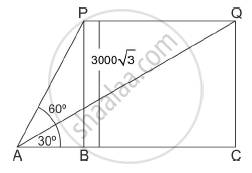# The angle of elevation of an aeroplane from a point on the ground is 60°. After a flight of 30 seconds the angle of elevation becomes 300 If the aeroplane is flying at a constant height of 3000 3 m, find the speed of the aeroplane. - Mathematics

The angle of elevation of an aeroplane from a point on the ground is 60°. After a flight of 30 seconds the angle of elevation becomes 300 If the aeroplane is flying at a constant height of 3000 3 m, find the speed of the aeroplane.

#### SolutionLet P and Q be the two positions of the plane and A be the point of observation. Let ABC be the horizontal line through A.
It is given that angles of elevation of the plane in two positions P and Q from a point A are 60° and 30° respectively.

∠PAB = 60°, ∠QAB = 30°. It is also given that PB = 3000√3 meters
In ΔABP, we have

tan 60="BP"/"AB"

sqrt3/1=(3000sqrt3)/"AB"

AB=3000m

In ΔACQ, we have

tan 30="QC"/"AC"

1/sqrt3=(3000sqrt3)/"AC"

AC = 9000 m
∴ Distance = BC = AC – AB = 9000m – 3000m = 6000m
Thus, the plane travels 6km in 30 seconds
Hence speed of plane = 6000/30 = 200 m/sec = 720km/h

Concept: Heights and Distances
Is there an error in this question or solution?